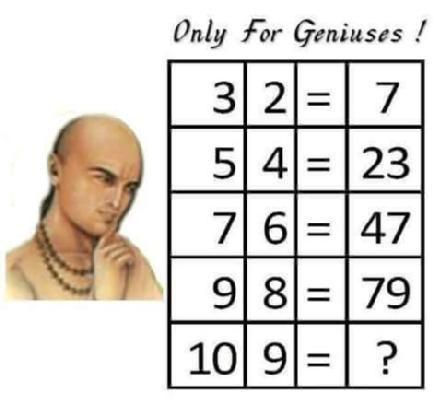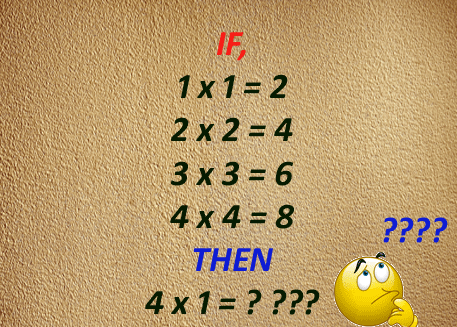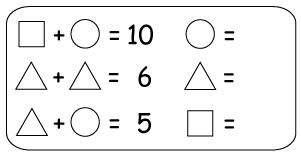#### Hello.

It looks like you're new here. If you want to get involved, click one of these buttons!

"Still lie the sheltering snows, undimmed and white;
And reigns the winter's pregnant silence still;
No sign of spring, save that the catkins fill,
And willow stems grow daily red and bright.
These are days when ancients held a rite
Of expiation for the old year's ill,
And prayer to purify the new year's will."
Helen Hunt Jackson, A Calendar of Sonnet's: February# For geniuses only. :)

edited October 2015 in Riddles
One name that comes across my mind is @Xanthippe who can easily solve it.• @amrtarekhassan Good job done.• edited October 2015
Let me share another one.• Hi @Bubbly , i like your riddles , this one is very easy or very difficult ,
But I think the answer is "5"
• Thank you @Rafiarema ...
• @Bubbly i think 99 for the first picture and 5 for the secuond picture
• let me share another one :smile ;)
s+m = 8
s-m = 4
d+c = 12
d-c = c
c+c = d
so:
s= ?
m= ?
d= ?
c= ?
• OMG! I hate math!(((
• @Glorian You may participate in activities other than intellectual puzzles
• @nisreen.mustafa nicely done.
• nicely done @nisreen.mustafa , you are a good competitor .. :wink:
• What on earth!
• The answer is 98 @bubbly
• @anjuz which one you talk about ??
• @Bubbly : 3*2+1=7, 5*4+3=23, 7*6+5=47, 9*8+7=79, 10*9+8=98.
Multiple the given number and add the result with less than one of the last number.
How 99 is the correct answer @amrtarekhassan
• edited October 2015
@anjuz +1, +3, +5, +7, +9. so It is 10*9+9 = 99.
• Dear @anjuz, it is easy measy , every step you add 2 to the difference .. So
3*2+1=7, 5*4+3=23, 7*6+5=47, 9*8+7=79, 10*9+9=99.
• Well Done @Rafiarema , you get it. :smile:
The answer is s=6, m = 2, d=8, c=4.
• Good jop @anjuz ,, well done :smile:
• @amrtarekhassan .ok that's it. But a solved it differently.
• @anjuz yes, well done :) ;)
• Here is the correct answer of first riddle. Well done guys.

3*2+1=7 5*4+3=23 7*6+5=47 9*8+7=79 10*9+9=99
@Rafiarema well done. You are good in maths.• @jawadamir Thank you for sharing this. Bring some more riddles.